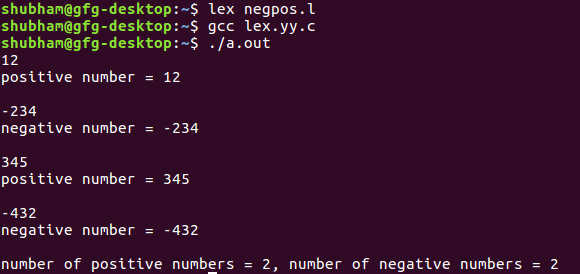GeeksforGeeks App
Open AppBrowser
Continue

# Lex Program to Identify and Count Positive and Negative Numbers

Given some numbers, task is to identify the positive and negative numbers and print the count of negative and positive numbers.

Prerequisite: Flex (Fast lexical Analyzer Generator)

Examples:

```Input : -52
Output :
negative number=-52
number of positive numbers = 0
number of negative numbers = 1

Input : 63
Output :
positive number = 63
number of positive numbers = 0
number of negative numbers = 1
```

Below is the implementation program:

 `/* Lex program to Identify and Count ``Positive and Negative Numbers */` `%{``int` `positive_no = 0, negative_no = 0;``%}``  ` `/* Rules for identifying and counting ``positive and negative numbers*/``%%``^[-][0-9]+ {negative_no++; ``            ``printf``(``"negative number = %s\n"``,``                  ``yytext);}  ``// negative number`` ` `[0-9]+ {positive_no++;``        ``printf``(``"positive number = %s\n"``, ``                 ``yytext);} ``// positive number     ``%% `` ` `/*** use code section ***/`` ` `int` `yywrap(){}``int` `main()                                              ``{``  ` `yylex(); ``printf` `(``"number of positive numbers = %d,"``        ``"number of negative numbers = %d\n"``,``                ``positive_no, negative_no);`` ` `return` `0; ``}`

Output:My Personal Notes arrow_drop_up